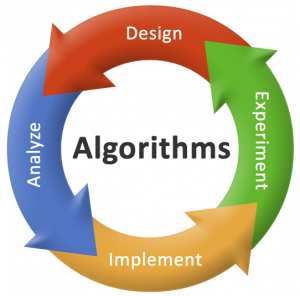# Analysis of Algorithms

In this tutorial you will learn about analysis of algorithms.

Before learning analysis of algorithms lets quickly take a look on what is an algorithm and why we require it.

### What is an Algorithm?

An algorithm is the step by step unambiguous procedure to solve a given problem.

For example steps for making Tea or Coffee can be considered as an algorithm.

So for solving a problem we require an algorithm.

## Analysis of AlgorithmsImage Source

There can be several algorithms for solving one problem. So analysis of algorithms is required to find the correctness (algorithm should give solution of problem in finite number of steps) and efficiency (time and memory it takes) of an algorithm.

For example: To go from city A to B there can be several ways like by bus, train, flight, etc.  Depending upon the availability and convenience we choose the one that suits best for us.

Similarly in computer science we use analysis of algorithms to choose the algorithm which is most efficient in terms of running time and space required.

### Running Time Analysis

It is the process of determining how processing time of an algorithm increase as the input size increase.

Input size is the number of elements in the input. There can be different types of inputs depending upon the problem type. Below are some common types of inputs.

• Size of an array.
• Number of elements in matrix.
• Vertices and edges in a graph.

### Types of Analysis

To analyze an algorithm we need to know for which input the algorithm takes less time and for which input it takes long time.

In terms of running time there can be three cases mentioned below.

Worst Case: It defines the input for which the algorithm takes longest time (slowest time) to complete.

Best Case: It defines the input for which the algorithm takes least time (fastest time) to complete.

Average Case: Run the algorithm using different random inputs and then find total running time by finding average of all running times.

Comment below if have any queries regarding above tutorial.